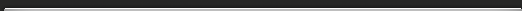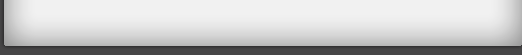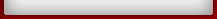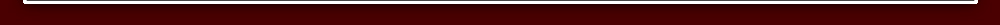Tutorial 3

## Tutorial 3

The easy way to add and subtract fractions.
Use VERTICALLY AND CROSSWISE to write the answer straight down!2 x 5 = 10 and 1 x 3 = 3. Then 10 + 3 = 13.
The bottom of the fraction is just 3 x 5 = 15.
You multiply the bottom number together.

So:Subtracting is just as easy: multiply crosswise as before, but the subtract:Exercise 1 Tutorial 3
Try a few: